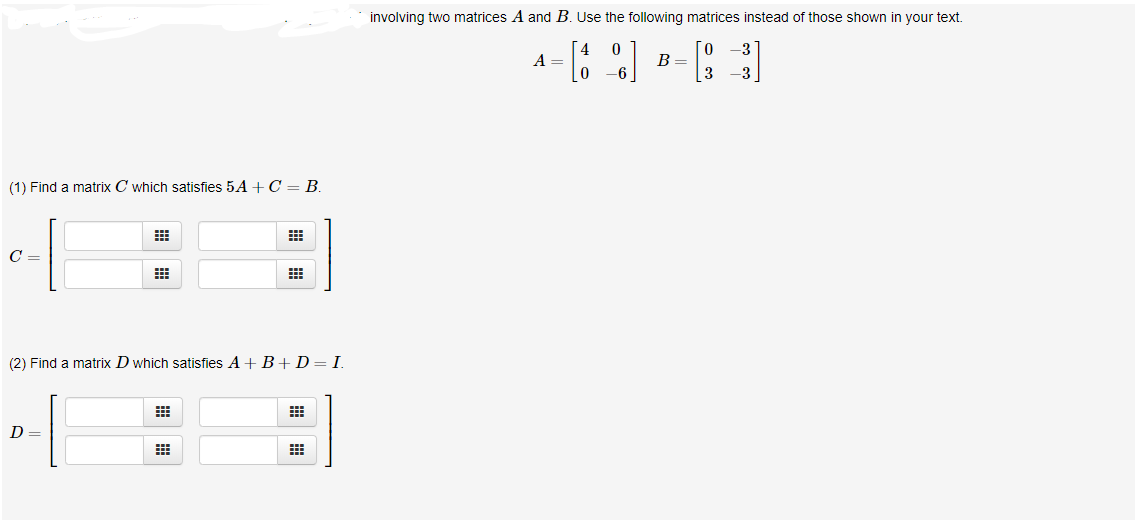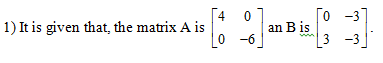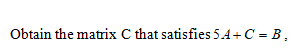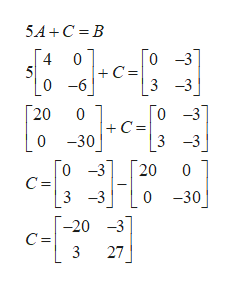# involving two matrices A and B. Use the following matrices instead of those shown in your text0-3А —A =B =33(1) Find a matrix C which satisfies 5 A C = BC =(2) Find a matrix Dwhich satisfies AB+D= I.

Question
1 viewshelp_outlineImage Transcriptioncloseinvolving two matrices A and B. Use the following matrices instead of those shown in your text 0 -3 А — A = B = 3 3 (1) Find a matrix C which satisfies 5 A C = B C = (2) Find a matrix Dwhich satisfies A B+D= I. fullscreen
check_circle

Step 1Step 2...help_outlineImage Transcriptionclose5A C B Го -31 C = 3 -3 0 5 0 -6 Го -37 C = 3 -3 20 0 0 -30 0 -3 20 0 C = 0 -30 3-3 -20 -3 C = 3 27 fullscreen

### Want to see the full answer?

See Solution

#### Want to see this answer and more?

Solutions are written by subject experts who are available 24/7. Questions are typically answered within 1 hour.*

See Solution
*Response times may vary by subject and question.
Tagged in

### Math